# Python数据结构与算法（一）——数据结构与算法基础、时间复杂度、列表与字典的时间复杂度685-杜同学

## 热门标签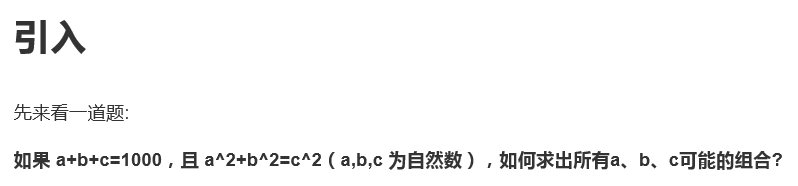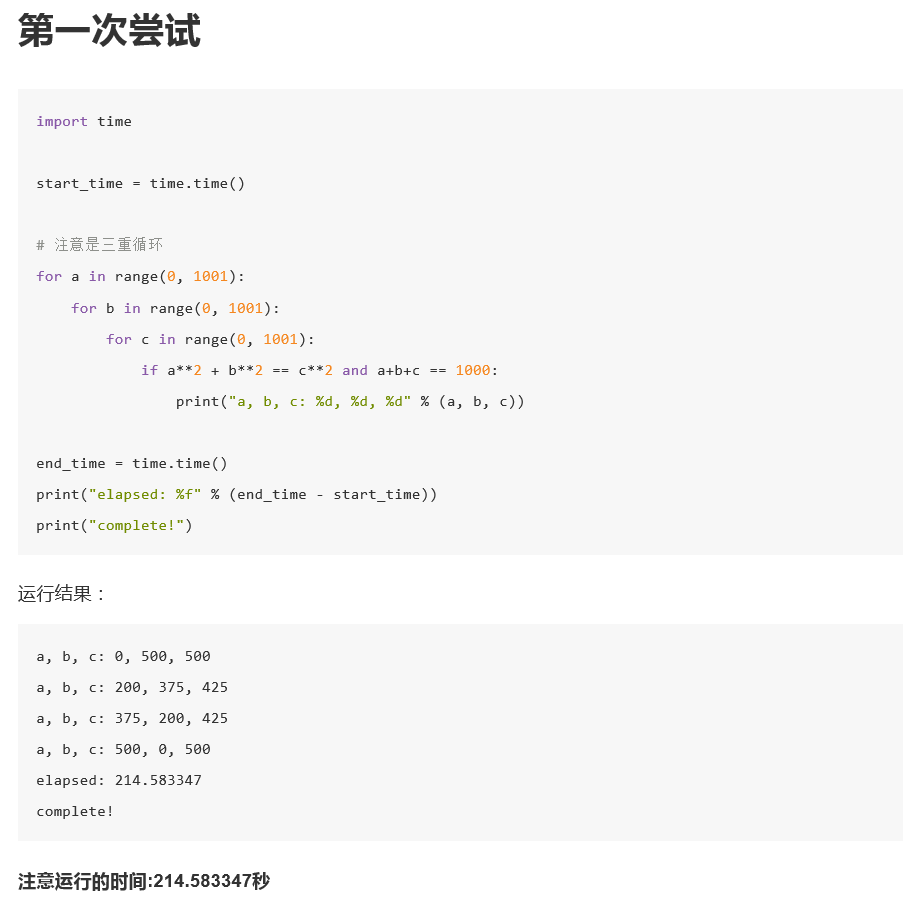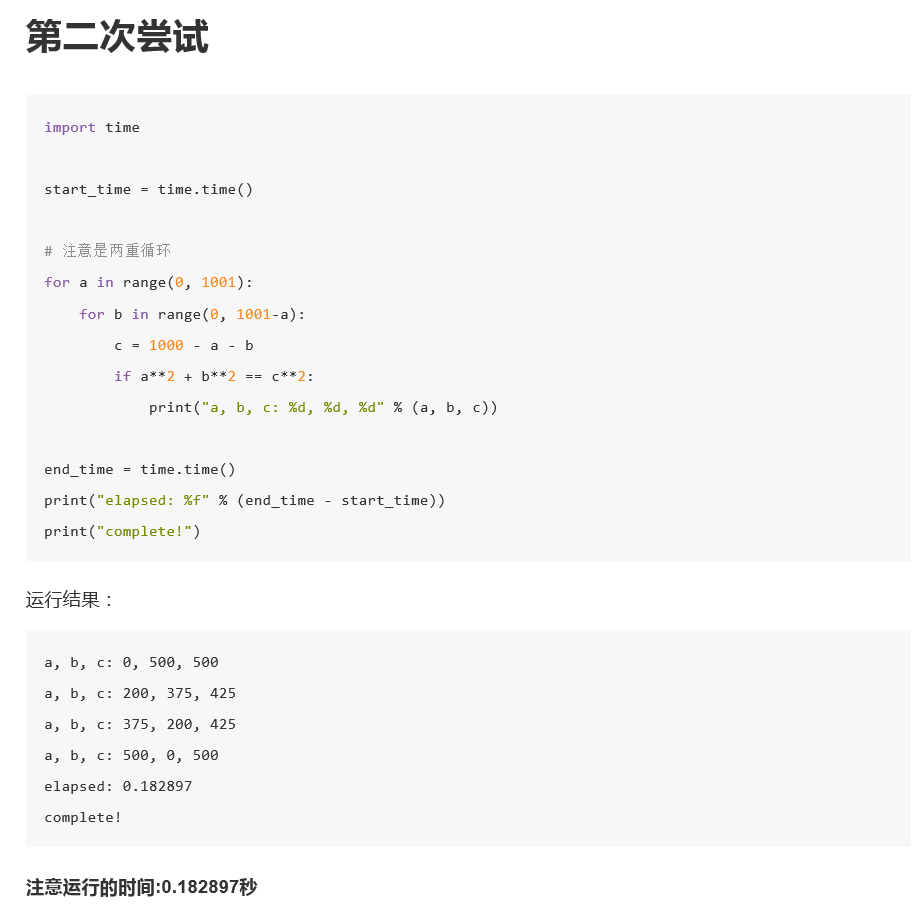## 时间复杂度的几条基本计算规则

1. 基本操作，即只有常数项，认为其时间复杂度为O(1)
2. 顺序结构，时间复杂度按加法进行计算
3. 循环结构，时间复杂度按乘法进行计算
4. 分支结构，时间复杂度取最大值
5. 判断一个算法的效率时，往往只需要关注操作数量的最高次项，其它次要项和常数项可以忽略
6. 在没有特殊说明时，我们所分析的算法的时间复杂度都是指最坏时间复杂度

​​​​​​​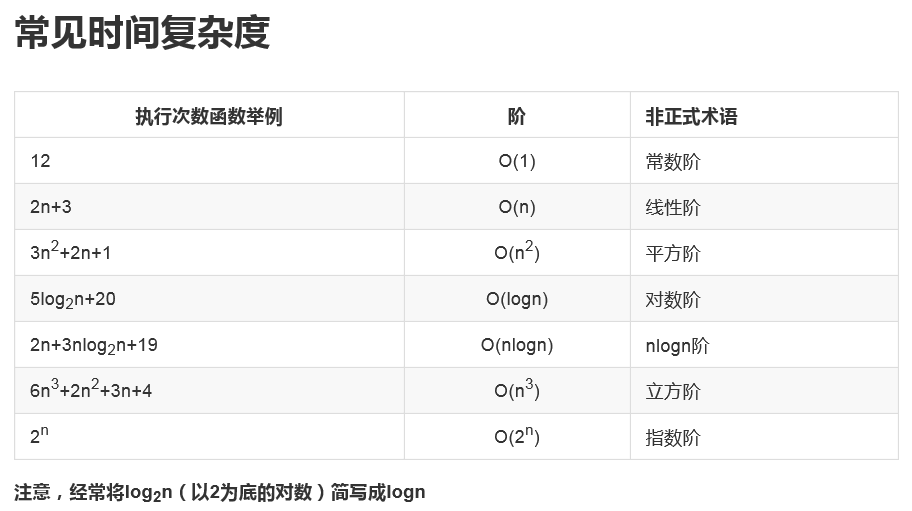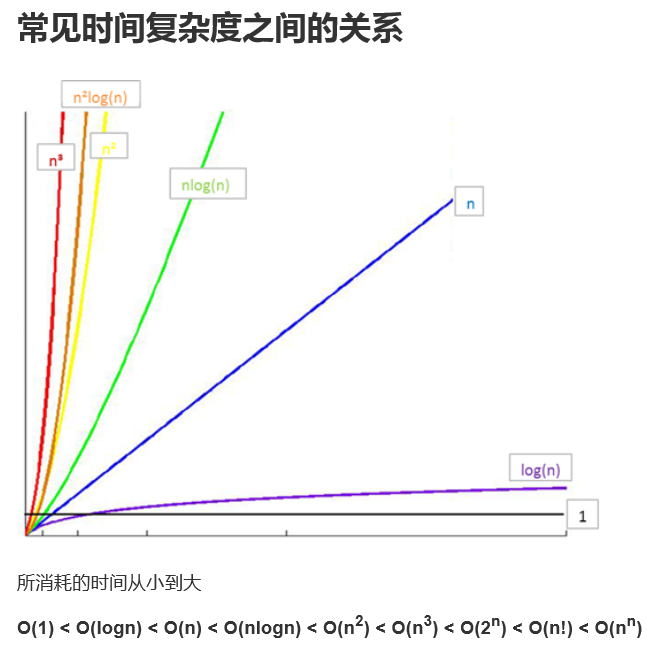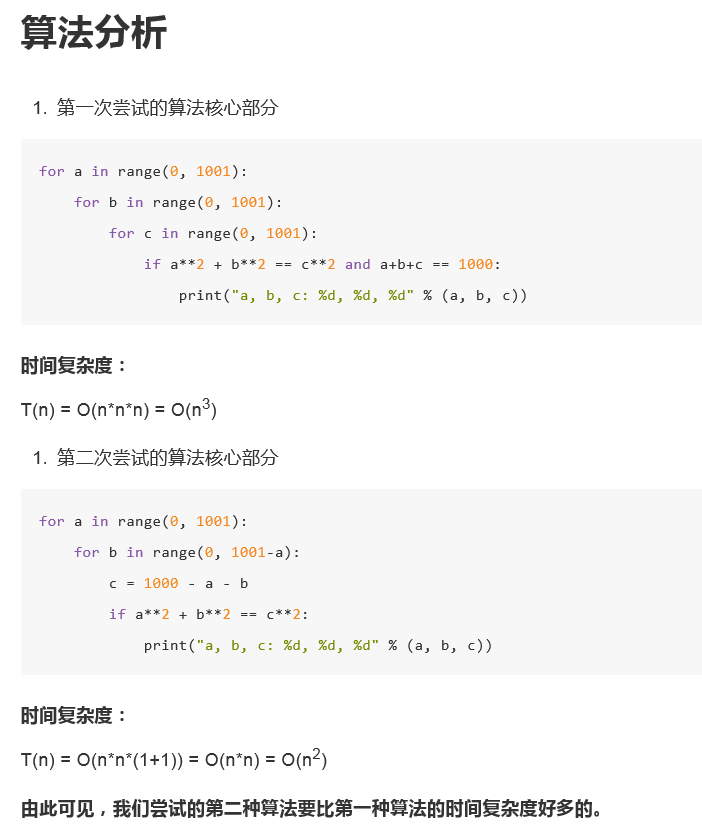## timeit模块

class timeit.Timer(stmt='pass', setup='pass', timer=<timer function>)

stmt参数是要测试的代码语句（statment）

setup参数是运行代码时需要的设置

timer参数是一个定时器函数，与平台有关。

timeit.Timer.timeit(number=1000000)

Timer类中测试语句执行速度的对象方法。number参数是测试代码时的测试次数，默认为1000000次。方法返回执行代码的平均耗时，一个float类型的秒数。

## list的操作测试

from timeit import timeit

def test1():
l = []
for i in range(1000):
l = l + [i]
def test2():
l = []
for i in range(1000):
l.append(i)
def test3():
l = [i for i in range(1000)]
def test4():
l = list(range(1000))

from timeit import Timer

t1 = Timer("test1()", "from __main__ import test1")
print("+ ",t1.timeit(number=1000), "seconds")
t2 = Timer("test2()", "from __main__ import test2")
print("append ",t2.timeit(number=1000), "seconds")
t3 = Timer("test3()", "from __main__ import test3")
print("comprehension ",t3.timeit(number=1000), "seconds")
t4 = Timer("test4()", "from __main__ import test4")
print("list range ",t4.timeit(number=1000), "seconds")

# ('+ ', 1.7890608310699463, 'seconds')
# ('append ', 0.13796091079711914, 'seconds')
# ('comprehension ', 0.05671119689941406, 'seconds')
# ('list range ', 0.014147043228149414, 'seconds')

pop操作测试

x = range(2000000)
pop_zero = Timer("x.pop(0)","from __main__ import x")
print("pop_zero ",pop_zero.timeit(number=1000), "seconds")
x = range(2000000)
pop_end = Timer("x.pop()","from __main__ import x")
print("pop_end ",pop_end.timeit(number=1000), "seconds")

# ('pop_zero ', 1.9101738929748535, 'seconds')
# ('pop_end ', 0.00023603439331054688, 'seconds')

### 数据结构

# 使用列表+元组存储
[
('Tom',24,'Beijing'),
('Jerry',23,'Shanghai'),
('Merry',24,'shenyang'),
]

# 使用列表加字典存储
[
{
'name':'Tom',
'age':24,
'hometown':'Beijing',
},
]

# 使用字典加字典存储
{
'Tom':{'age':24,'hometown':'Beijing'},
'Jerry':{'age':23,'hometown':'Shanghai'},
'Merry':{'age':24,'hometown':'Shenyang'}
}

### Python中内置的数据结构

list内置操作的时间复杂度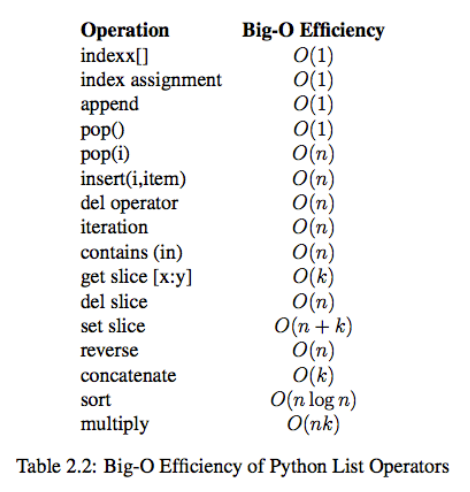dict内置操作的时间复杂度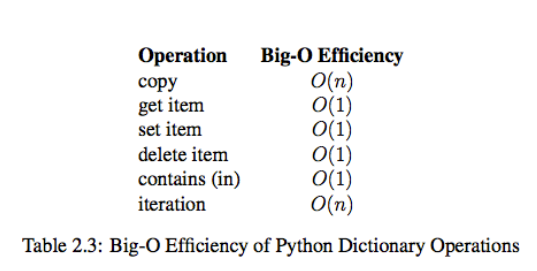• 插入
• 删除
• 修改
• 查找
• 排序

### 评论 抢沙发### 觉得文章有用就打赏一下文章作者

#### 支付宝扫一扫打赏#### 微信扫一扫打赏Vieu3.3主题Q Q 登 录